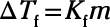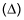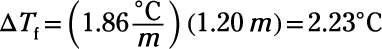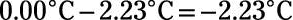##### Chemistry Workbook For Dummies with Online PracticeAn important property that you can calculate from molality is freezing point depression. When you add solute to a solvent, it lowers its freezing point. That's why you sprinkle salt on icy sidewalks. The salt mixes with the ice and lowers its freezing point.

If this new freezing point is lower than the outside temperature, the ice melts, eliminating the spectacular wipeouts so common on salt-free sidewalks. The colder it is outside, the more salt you need to melt the ice and lower the freezing point to below the ambient temperature.

Freezing point depressions are directly proportional to the molality of a solution, but chemists have found that some solvents are more susceptible to this change than others. The formula for the change in the freezing point of a solution, therefore, contains a proportionality constant, abbreviated Kf, which is a property that is determined experimentally. The table lists several common Kf values.

Common Kf Values
Solvent Kf in Degrees C/m Freezing Point in Degrees C
Acetic acid 3.90 16.6
Benzene 5.12 5.5
Camphor 37.7 179.0
Carbon tetrachloride 30.0 –23.0
Cyclohexane 20.2 6.4
Ethanol 1.99 –114.6
Phenol 7.40 41.0
Water 1.86 0.0
The formula for the freezing point depression iswhere m is molality. Note the use of the Greek letter deltain the formula to indicate that you're calculating a change in the freezing point, not the freezing point itself. To calculate the new freezing point of a compound, you must subtract the change in freezing point from the freezing point of the pure solvent.

Adding an impurity to a solvent alters its physical properties through the combined effects of boiling point elevation and freezing point depression. That's why you rarely see bodies of frozen salt water. The salt in the oceans lowers the freezing point of the water, making the liquid phase able to sustain temperatures slightly below 0 degrees Celsius.

Here's an example: each kilogram of seawater contains roughly 35 g of dissolved salts. Assuming that all these salts are sodium chloride, what is the freezing point of seawater?

Begin by converting grams of salt to moles to figure out the molality. One mole of NaCl is equivalent to 58.4 g, so 35 g is equivalent to 0.60 mol of NaCl. You need to multiply this number by 2 to compensate for the fact that sodium chloride dissociates into two ions in water, so this solution contains 1.20 mol of particles.

Next, find the molality of the solution by dividing this number of moles by the mass of the solvent (1 kg), giving a 1.20 m solution. Last, look up the Kf of water in the table and plug all these values into the equation for freezing point depression:Because this value is merely the freezing point depression, you must subtract it from the freezing point of the pure solute to getSo, the freezing point of seawater is –2.23 degrees Celsius.